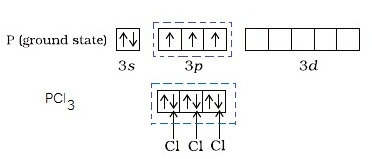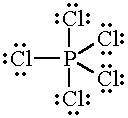# Question #039d7

Jul 9, 2017

The valency of phosphorus is (3.) 3 and 5.

#### Explanation:

Many chemists define the valency of an element as the number of hydrogen or chlorine atoms that it can combine with.

Phosphorus is in Group 15 of the Periodic Table, so its electron configuration is ${\text{[Ne] 3s"^2 "3p}}^{3}$.

It therefore has five valence electrons.In the orbital diagram above, the three unpaired electrons in the $\text{3p}$ orbitals can form bonds to three $\text{Cl}$ atoms and form ${\text{PCl}}_{3}$.In this case, the valency of phosphorus is three.

Another possibility is that that $\text{P}$ might "promote" a $\text{3s}$ electron to a $\text{3d}$ orbital.The atom would then have five unpaired electrons, so it could form bonds to five $\text{Cl}$ atoms and form ${\text{PCl}}_{5}$.In this case, the valency of phosphorus is five.

Thus, the valency of phosphorus is three and five.